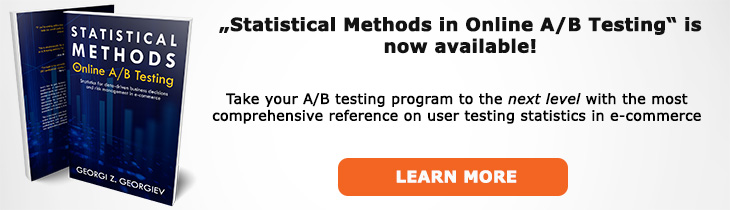# What does "Significance Test" mean?

Definition of Significance Test in the context of A/B testing (online controlled experiments).

## What is a Significance Test?

Aliases: Test of Significance

A significance test is a statistical procedure in which a null hypothesis is tested using a distance measure with a known probability distribution and a particular value of the distance measure is obtained. Most often in A/B testing the distance measure takes the form of a z score or t score which is compared to a specified rejection region. Based on where it falls: inside or outside of the region the null hypothesis is rejected or not. Significance tests are a crucial part of causal inference in online controlled experiments.

Often the result of the test is communicated directly in the form of a p-value: the probability of observing an outcome as extreme or more extreme than the observed, under the assumption the null hypothesis is true.

Usually an extension of the procedure in which an alternative hypothesis is introduced which complements the null and is accepted if the null is rejected and can be rejected (to an extent) otherwise. In such a case a significance test can be expressed formally as the following decision rule: if p(x0) ≤ α, reject H0 (infer H1); if p(x0) > α, do not reject H0, where alpha is the significance threshold and H0 is the null, H1 is the alternative.

Crucially, in order for the data to be correctly assessed by a significance test it has to be performed under an adequate statistical model. Misspecification / violations of the test's assumptions inevitably lead to more or less severe departures from its accuracy, sometimes to the point of losing its meaning entirely.

Due to known fallacies in interpretation of the outcomes of tests of significance and confidence intervals alternative approaches such as severity testing are advanced by some current philosophers of science and statisticians.

## Articles on Significance Test

Like this glossary entry? For an in-depth and comprehensive reading on A/B testing stats, check out the book "Statistical Methods in Online A/B Testing" by the author of this glossary, Georgi Georgiev.## Glossary Index by Letter

Select a letter to see all A/B testing terms starting with that letter or visit the Glossary homepage to see all.